# SSD模型详解

SSD网络总体描述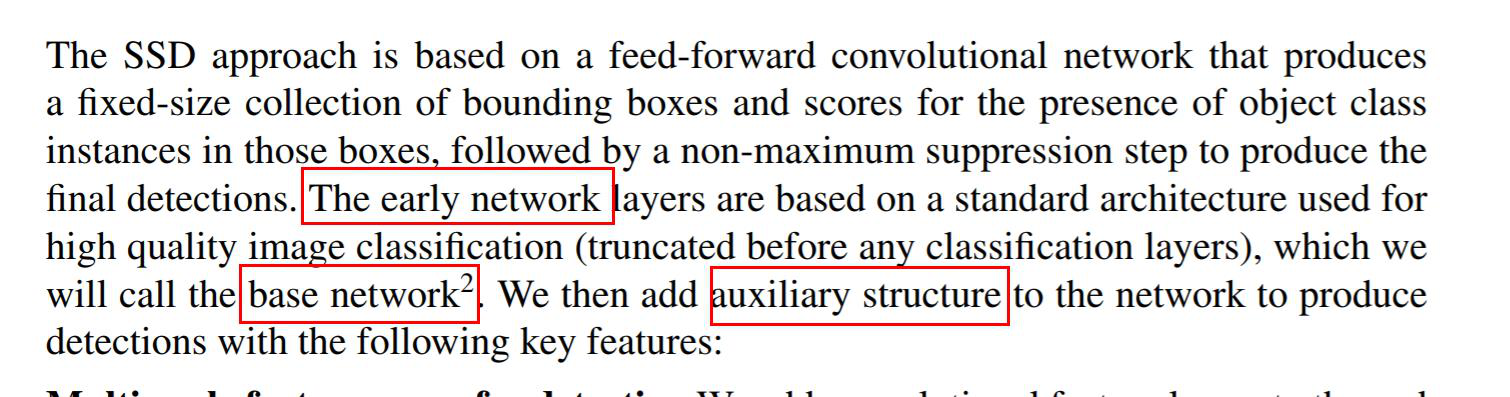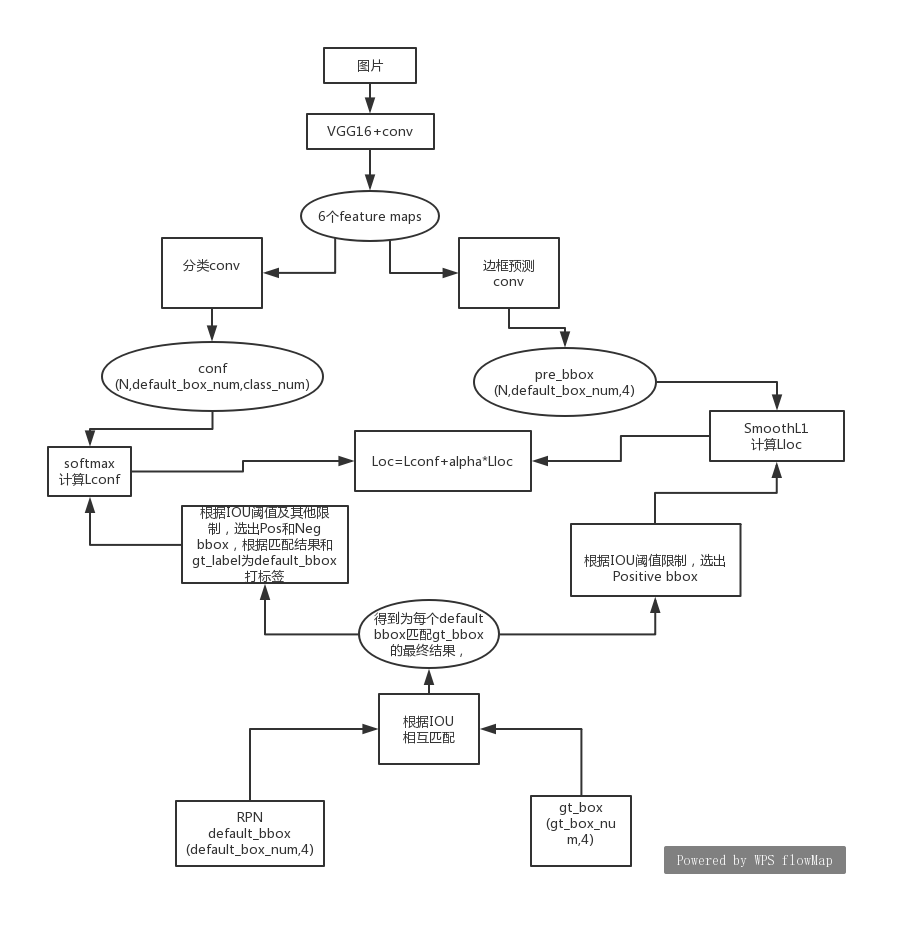1.基础网络 (base network)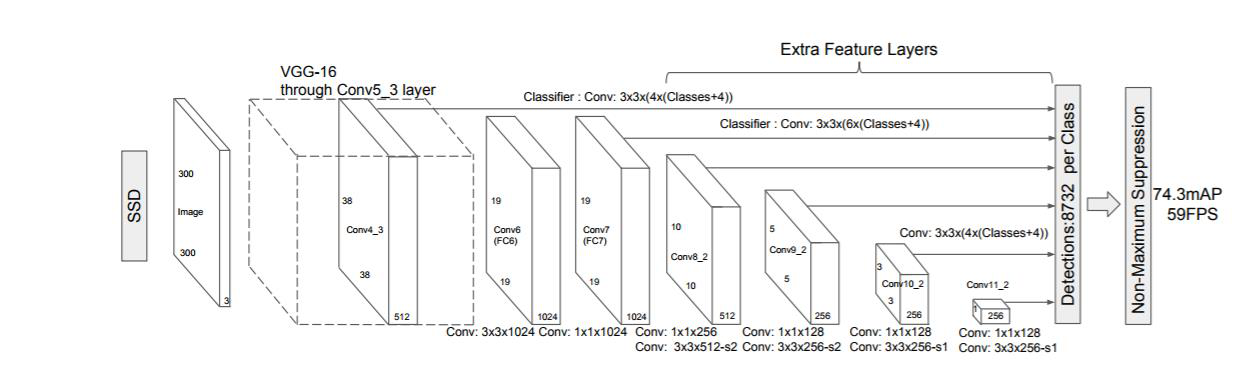2.分类网络和边框预测网络

bbox的四个输出值含义分别为（与相对应的default box的中心x坐标偏移，中心y坐标偏移，width偏移值，height偏移值）

total number of prediction bounding box的计算过程（设定在RPN中为第一个feature map为每个pixel生成3个default box（论文中是6个），其余各层6个）
Feature map1:（N,512,19,19） 边框预测网络conv层filters数3x4
Feature map2：（N,1024,10,10） filters num 6x4
Feature map2:（N,512,5,5） filters num 6x4
Feature map3：（N,256,3,3） filters num 6x4
Feature map4：（N,256,2,2） filters num 6x4
Feature map5：（N,128,1,1） filters num 6x4

      """num_bboxes为用RPN计算得到的每个pixel的default box的数目,在ssd中
为6，在边框预测网络中就将卷积核数目设置成6*4,kernel_size和stride
都设为1，所以卷积输出的channel是num_bboxes*4，每个channel的width和
height不变
因为该conv层最终输出shape：(N,num_bboxes,width,height)
含义为输入feature map的每个pixel都生成num_bboxes个预测边框，4代表预测边框相对于
default box的偏移值"""
num_loc_output = num_boxes * 4
mbox_loc = nn.conv2d(
input=input,
num_filters=num_loc_output,
filter_size=kernel_size,
padding=pad,
stride=stride)
#(N,num_loc_output,width,height)reshape成
#(N,width,height,num_loc_output)
mbox_loc = nn.transpose(mbox_loc, perm=[0, 2, 3, 1])
#将其展平成2d矩阵（N,width*height*num_loc_output)
mbox_loc_flatten = nn.flatten(mbox_loc, axis=1)
#将当前feature map运算结果存入列表中，最终将6个flatten的结果
#concat成一个矩阵
mbox_locs.append(mbox_loc_flatten)


3.生成Default Bbox

height不一样。
Default box的中心坐标可以根据上图红框中最后一个公式算出。

（minx,miny,maxx,maxy）这些坐标都是比例值，被规范在(0,1)之间，将计算得到的bounding box映射到原图像中，在原图像中画出这些边框，下面是简化代码

import numpy as np
import cv2
import matplotlib.pyplot as plt
import math

#计算feature map的sk
def s_size(k=1):
smin=0.2
smax=0.9
m=6
ssize=smin+(smax-smin)/(m-1)*(k-1)
return ssize

#根据feature map的sk和aspect ratio计算width和height
def w_h(ssize,k):
width = []
height = []
aspect_ratio = [1,2,3,1/2,1/3]
ssize2 = s_size(k+1)
ssize2 = math.sqrt(ssize2*ssize)
width.append(ssize2*math.sqrt(aspect_ratio))
height.append(ssize2/math.sqrt(aspect_ratio))
for i in range(len(aspect_ratio)):
width.append(ssize*math.sqrt(aspect_ratio[i]))
height.append(ssize/math.sqrt(aspect_ratio[i]))
return width,height

def default_box(fk,k):
#为每个pixel生成6个default box
#因为卷积操作，实则是映射到原图上生成size不同的bbox的
ssize = s_size(k)
widths,heights =  w_h(ssize,k)
bboxes = []
#为第k个feature map的fk*fk的pixel生成6个default box
for i in range(fk):
for j in range(fk):
center_x = (i+0.5)/fk
center_y = (j+0.5)/fk
bboxij=[]
for num in range(len(widths)):
box = []
#xmin,ymin,xmax,ymax
box.append(max(0, center_x - widths[num]/2))
box.append(max(0, center_y - heights[num]/2))
box.append(min(1, center_x + widths[num]/2))
box.append(min(1, center_y + heights[num]/2))
bboxij.append(box)
bboxes.append(bboxij)

#以此坐标看画出来的6个bbox以哪个位置为准，
#在下面画出第len(bboxes)个bboxes
#if i==4 and j==4:
#    print(len(bboxes))
#bboxes[i*j]
return bboxes

def main():
img = cv2.imread('./pic2.jpg')
pic = cv2.resize(img,(500,500),interpolation=cv2.INTER_AREA)
#10是feature map的单边尺寸,第3个feature map
bboxes = default_box(10,3)
for i in range(10*10):
if i==45:
w=pic.shape
for num in range(6):
x1 = int((bboxes[i][num])*w)
y1 = int(bboxes[i][num]*w)
x2 = int(bboxes[i][num]*w)
y2 = int(bboxes[i][num]*w)
cv2.rectangle(pic, (x1, y1), (x2, y2), (255, 0, 0), 2)
cv2.imshow('test',pic)
cv2.waitKey(0)
return

if __name__ == '__main__':
main()


39x39的feature map中计算出的default bbox，一共生成了39x39x6个边框，从图中可以看出边框size比较小，框不住大牛。但是小牛完全可以框住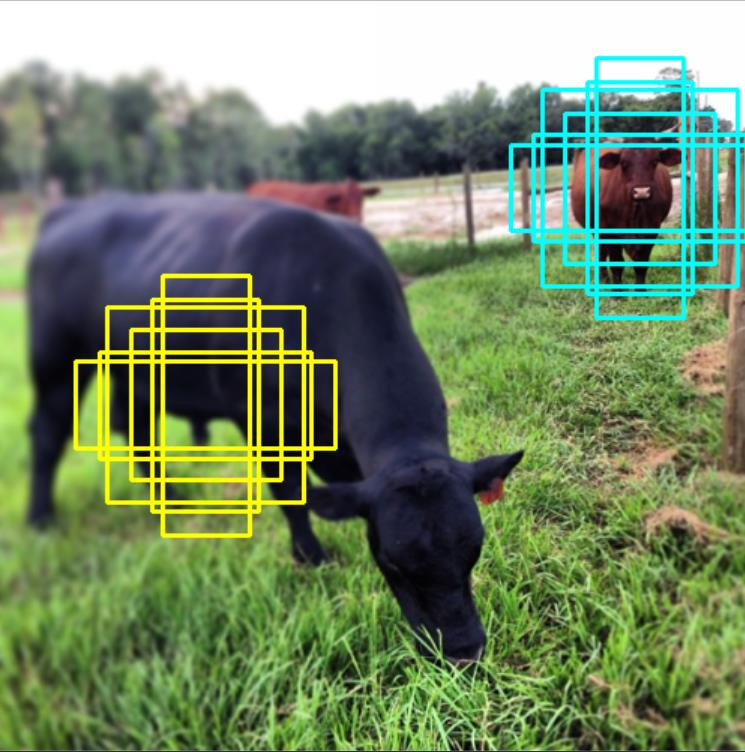下面这张图是feature map的shape为（10,10）的情况下生成的边框，可以框住相对比较大的object，所以对于大的object，需要计算出更大的default bbox，并用边框预测网络不断调整default bbox与gt_bbox的差距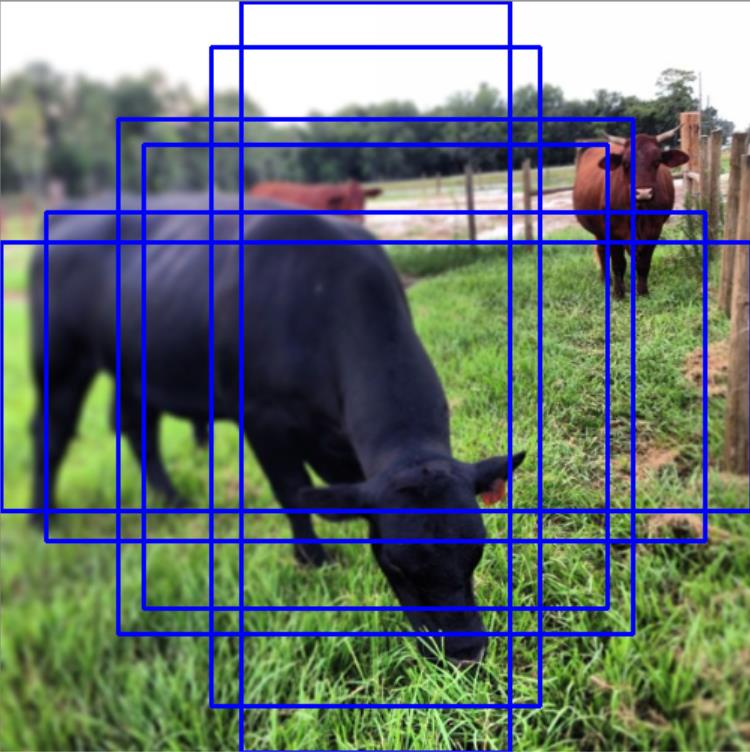4.目标函数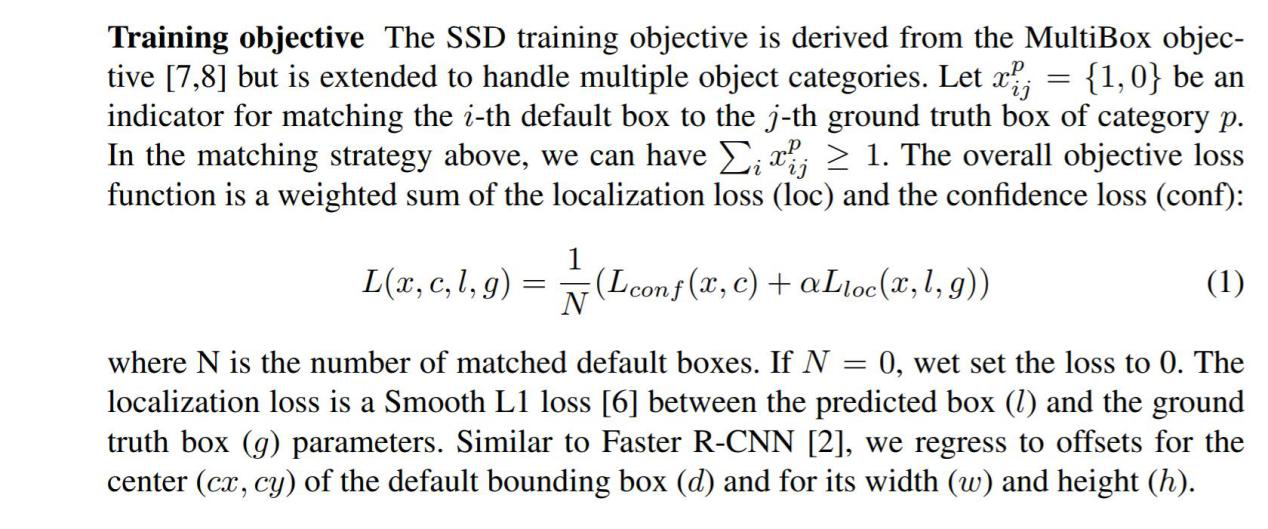下面是详细的公式，这两部分loss的计算都与default bbox和ground truth bbox的匹配有关，其中li是prediction bbox，g部分是为每个default_bbox匹配的ground truth bbox结果，d部分是default bbox，cx是bbox中心x坐标,cy是bbox中心y坐标。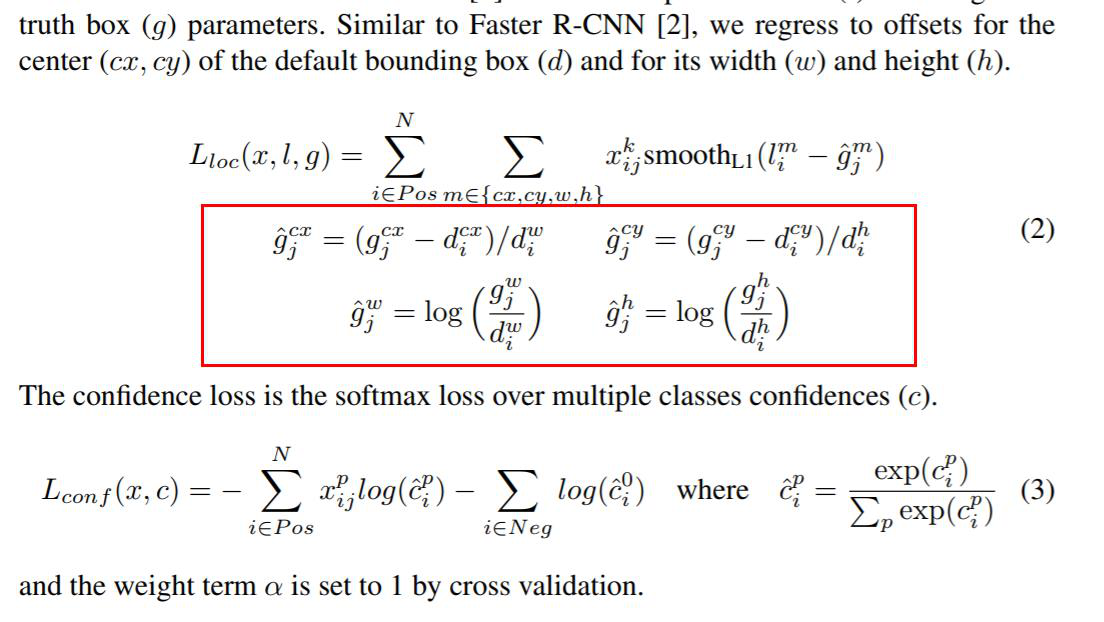通过分析ssd源码，简化理解default bbox与ground truth box的匹配

df_bbox: (df_num,4) 因为default bbox每张图片可共用
gt_bbox shape: (N,gt_num,4) 假设每张图片的真实object数一致

for i in range(N):
1、计算出df_bbox与gt_bbox(i)之间的IOU矩阵
iou的shape可为(gt_num,df_num),即(3,1917)

2、根据iou计算出与gt_bbox匹配的df_bbox的边框序号
即找出iou矩阵中每一行的最大值,得出它的列号。
匹配得到的索引list为df_index，长度为3

3、根据iou计算出与每个df_bbox匹配的gt_bbox的边框序号,则每个gt_bbox
都有可能被多次匹配到。
做法是选取iou矩阵中每一列的最大值的行号。
匹配得到的索引list为gt_index，长度为1917

4、保证第2、3步匹配到的结果是相互的，如第1个gt_bbox匹配到的df_bbox是第
200个，第200个df_bbox匹配到的gt_bbox也是第1个，如不相符合，以第2步的匹配为准

5、根据第3步得到的gt_index,得到df_bbox对应于gt_bbox的匹配矩阵
即match=gt_bbox[gt_index]，它的shape为 (1917,4),和df_bbox是
一致的，这个match就是论文公式中的g部分。

6、根据match、df_bbox计算上面图片中红框框出的一部分，代表的含义是
每个df_bbox相对于匹配到的gt_bbox的中心坐标偏移距离，以及width和
height偏移。[ lg(a/b) = lg(a) - lg(b) ]
计算结果为loc_enc，shape为(1917,4)

7、在df_box中肯定有很多边框是背景边框,所以根据设定的iou阈值,选出用于计算Lloc损失
中的Positive bbox,iou阈值可设为0.5。只选择计算Positive边框的损失。

8、Lloc公式中的li值是网络的prediction值，值的含义是相对df_bbox的四个值
的偏移，df_bbox的值本身是固定的，所以通过不断调整网络的prediction偏移值，就
相当于不断调整df_bbox，使之不断逼近gt_bbox。


pre_bbox: (N,pre_num,class_num) pre_num等于default bbox数目
gt_label: (N,gt_num) 假设每张图片的真实object数一致

for i in range(N):
1、为prediction bbox打标签,根据上面计算Lloc过程中匹配得到的gt_index
pre_label = gt_label[gt_index]

2、设定iou阈值，将给df_bbox匹配gt_bbox的结果中iou小于阈值的边框
设为Negative bbox,在此处是将其pre_label设置为0，即代表背景

3、很大一部分df_box都可能被判定为背景，这样会导致负例太多,正负比例不均,所以需要调整正负比,
可选择Pos:Neg=1:3 选择方法可以为：首先根据步骤2中的Neg和Pos计算出
loss,再根据正负例比例,从中选出loss比较大的几个Neg边框（不确定是
loss是较大还是较小，但感觉上取较大的比较合理）

4、接下来就根据选出的Pos和Neg边框,计算交叉熵损失就得到Lconf了。


5.augmentation 数据增广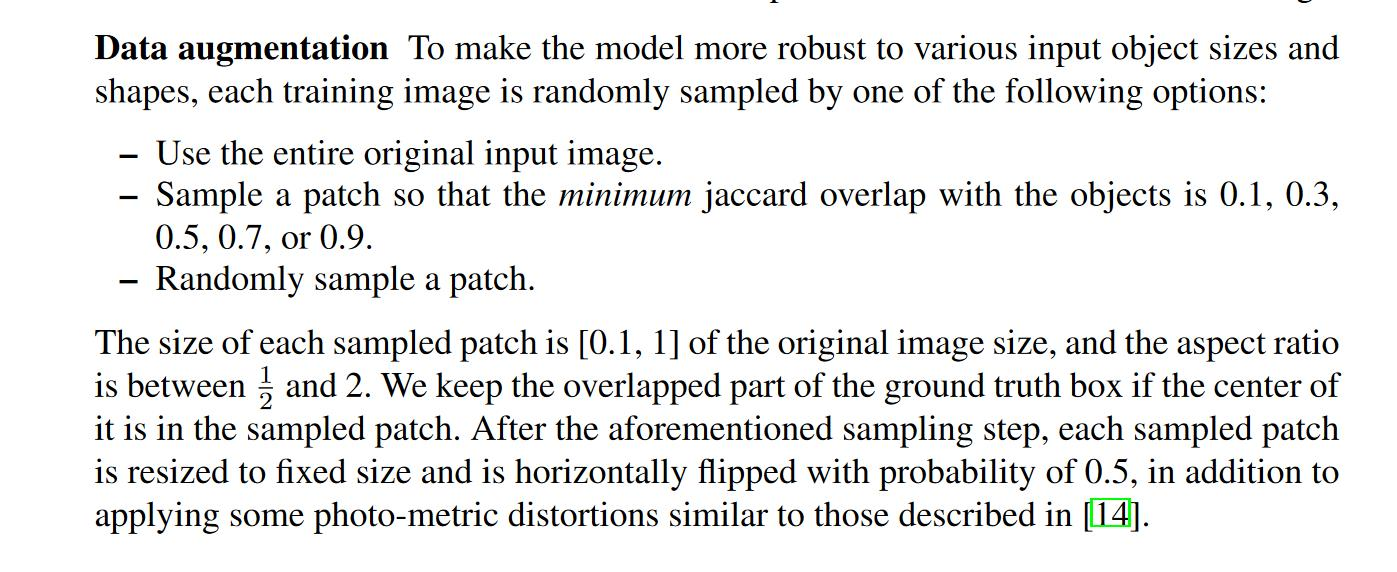ssd论文中数据增广的一部分过程,通过下面的函数理解

sampler(max_sampl=1, max_trial=50, min_scale=0.3, max_scale=1,
min_aspect_ratio=0.5, max_aspect_ratio=2, min_jaccard_overlap=0.1,
max_jaccard_overlap=0)

,根据scale和aspect_ratio计算出随机截取part的坐标、width、height。并且一共尝试50次，每个随机生成的part都要与原图像中的每个gt_bbox进行jaccard比较，看其jaccard值是否在[min_jaccard_overlap,max_aspect_ratio]范围内，只要随机part

6.base network中的两个细节小点
（1）基础网络（vgg-16）可以替换成其他的，比如MobileNet等，会相对轻量级一点。

（2）为什么要将VGG的全连接层改成卷积层且在其后增加了几个卷积层10-161011
05-273万+
07-304377
03-271588
08-114934
08-155万+
08-105498
05-304378
©️2020 CSDN 皮肤主题: 大白 设计师:CSDN官方博客